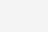# EasyX实现简易贪吃蛇

EasyX实现简易贪吃蛇@TOC 前言 我们曾经都玩过贪吃蛇游戏，所以我今天学习了C语言就想学习然后写一下。 一、头文件包含 包含完头文件后进行一些预处理操作： 二、创建蛇与食物的结构体 贪吃蛇是基于帧驱动，而不是事件驱动的📝个人主页：认真写博客的夏目浅石. 📣系列专栏：夏目的C语言宝藏

@TOC

# 一、头文件包含

``````#include<stdio.h>
#include<graphics.h> //图形处理需要包含的库文件
#include<mmsystem.h> //windows多媒体接口
#include<conio.h> //读取键盘输入
#include<time.h> //随机数种子
#include<stdlib.h> //内存分配
``````

``````#pragma comment(lib,"winmm.lib")//多媒体接口
#define NUM 200;
#define SIZE 10
enum Ch{ up=72,down=80,left=75,right=77} ;
``````

# 二、创建蛇与食物的结构体

``````struct pos //蛇身坐标 {
int x;
int y;
};
struct snake {
int n;    //初始化蛇身长度
Ch ch;    //蛇前进的方向
pos szb;  //蛇的坐标数组
};
struct food {
int x;
int y;
bool iseat;  //判断食物是否被吃
};

//创建一个蛇与食物

snake s;
food  f;
``````

# 三、游戏的初始化

``````void gameinit()
{
srand((unsigned int)time(NULL));//随机数种子，用于随机生成食物
s.n = 2;  //蛇的初始长度，可以自己设定
s.ch = right;//蛇的初始方向；
s.szb.x=100;
s.szb.y=100;
s.szb.x = 90;
s.szb.y = 100;//蛇的初始位置坐标
f.iseat = true;
}
``````

# 四、游戏的绘画事件

``````cleardevice();  //每次绘画都要进行一次清屏
setfillcolor(RED); //蛇头颜色
fillrectangle(s.szb.x, s.szb.y,
s.szb.x + SIZE, s.szb.y + SIZE);//SIZE在预处理是已经定义表示每个蛇身的大小
for (int i = 0; i < s.n; i++) {
rectangle(s.szb[i].x, s.szb[i].y,
s.szb[i].x + SIZE, s.szb[i].y + SIZE);
} //生成矩形蛇
setfillcolor(GREEN);//食物颜色
fillroundrect(f.x, f.y, f.x + SIZE, f.y + SIZE,5,5);//生成圆形食物
``````

# 五、蛇的移动事件

``````{
for (int i = s.n - 1; i > 0; i--)
{
s.szb[i].x = s.szb[i - 1].x;
s.szb[i].y = s.szb[i - 1].y;  //通过数组的变换来实现蛇的移动
}
switch (s.ch)//ch枚举类型在预处理时已经定义
{
case up:
s.szb.y -= SIZE;

//由于在窗口坐标系中y方向是向下的所以向上移动需要减去一个size
break;
case down:
s.szb.y += SIZE;
break;
case left:
s.szb.x -= SIZE;
break;
case right:
s.szb.x += SIZE;
break;
}
}

``````

# 六、输入方向

``````void changech()
{
char key;
key = _getch();
switch (key)
{
case up:
if (s.ch != down)
s.ch = up;
break;
case down:
if (s.ch != up)
s.ch = down;
break;
case left:
if (s.ch != right)
s.ch = left;
case right:
if (s.ch != left)
s.ch = right;
}
}

``````

# 七、生成食物

``````void creatfood()
{
bool flag = false;
if (f.iseat == true) {
while (1)
{
flag = false;//设定flag判断食物是否与蛇身重合
f.x = rand() % 68*10;
f.y = rand() % 48*10;//食物的随机生成
for (int i = 0; i < s.n; i++)
{
if (f.x == s.szb[i].x && f.y == s.szb[i].y)
{
flag = true;
break;
}
}
if (!flag)
{
f.iseat = false;
break;
}

//上面部分为判断食物是否与蛇身重合
}
}
}
``````

# 八、吃食物

``````void eatfood()
{
if (s.szb.x == f.x && s.szb.y == f.y)//如果蛇头与食物重合那么食物就被吃掉了
{
f.iseat = true;
s.n++;
}
}
``````

# 九、游戏失败的判定

``````int gameover()
{
if (s.szb.x < 0 || s.szb.x>640 || s.szb.y < 0 || s.szb.y>480)
{
return 1;
}
for (int i = 1; i < s.n; i++)
{
if (s.szb.x == s.szb[i].x && s.szb.y == s.szb[i].y)
{
return 1;
}
}
return 0;
}
``````

# 十、main函数

``````int main()
{
initgraph(680,480);
mciSendString(L"open 7895.mp3", 0, 0, 0);
mciSendString(L"play 7895.mp3", 0, 0, 0);

//添加音乐必须加上这个预处理指令#pragma comment(lib,"winmm.lib"）
int n = 0;
gameinit();
while (1) {
while (!_kbhit())
{
snakemove();
drawgame();
creatfood();
eatfood();
n = gameover();
if (n)
break;
Sleep(100);
}
if (n)
break;
changech();
}
closegraph();
getchar();
return 0;
}
``````

# 总结

(0)

### 相关推荐

• #### 一文彻底读懂MySQL事务的四大隔离级别

一文彻底读懂MySQL事务的四大隔离级别事务，由一个有限的数据库操作序列构成，这些操作要么全部执行,要么全部不执行，是一个不可分割的工作单位。 原子性： 事务作为一个整体被执行，包含在其中的对数据库的操作要么全部都执行，要么都不执行。 一致性： 指在事务开始之前和事务结束以后，数据不会被破坏，假如A账户给B账户转10…

• #### 数据库两大神器【索引和锁】[通俗易懂]

数据库两大神器【索引和锁】[通俗易懂]索引和锁在数据库中可以说是非常重要的知识点了，在面试中也会经常会被问到的。 表经常进行INSERT/UPDATE/DELETE操作就不要建立索引了，换言之：索引会降低插入、删除、修改等维护任务的速度。 索引需要占物理和数据空间。 为什么说索引会降低插入、删除、修改等维护任务的速…

• #### 云计算核心技术剖析（云计算使用的主要技术）

云计算核心技术剖析（云计算使用的主要技术）

• #### mysql安装（mysql8.0.34安装教程）

mysql安装（mysql8.0.34安装教程）

• #### scripting.filesystemobject（script文件）

scripting.filesystemobject（script文件）

• #### 平衡二叉树（平衡二叉树和完全二叉树的区别）

平衡二叉树（平衡二叉树和完全二叉树的区别）

• #### 面试官问我MySQL调优，我真的是「建议收藏」

面试官问我MySQL调优，我真的是「建议收藏」面试官：要不你来讲讲你们对MySQL是怎么调优的？ 候选者：哇，这命题很大阿…我认为，对于开发者而言，对MySQL的调优重点一般是在「开发规范」、「数据库索引」又或者说解决线上慢查询上。 候选者：而对

• #### 一种基于Redisson实现简单的分布式定时任务执行方案

一种基于Redisson实现简单的分布式定时任务执行方案一般在springcloud下单机执行定时任务的代码 但因为微服务下一般是多实例部署，会导致定时任务多个实例同时执行的情况。 通过对EnableScheduling进行分析，在调用定时方法时会把Met